Learn data science and machine learning by building real-world projects on Jovian
. 20 weeks part-time, 7 courses, 12 assignments, 4 projects, and 6 months of career support.

## Training Deep Neural Networks on a GPU with PyTorch

#### Part 4 of "Deep Learning with Pytorch: Zero to GANs"

This tutorial series is a hands-on beginner-friendly introduction to deep learning using PyTorch, an open-source neural networks library. These tutorials take a practical and coding-focused approach. The best way to learn the material is to execute the code and experiment with it yourself. Check out the full series here:

This tutorial covers the following topics:

• Creating a deep neural network with hidden layers
• Using a non-linear activation function
• Using a GPU (when available) to speed up training
• Experimenting with hyperparameters to improve the model

#### How to run the code

This tutorial is an executable Jupyter notebook hosted on Jovian. You can run this tutorial and experiment with the code examples in a couple of ways: using free online resources (recommended) or on your computer.

##### Option 1: Running using free online resources (1-click, recommended)

The easiest way to start executing the code is to click the Run button at the top of this page and select Run on Colab. Google Colab is a free online platform for running Jupyter notebooks using Google's cloud infrastructure. You can also select "Run on Binder" or "Run on Kaggle" if you face issues running the notebook on Google Colab.

##### Option 2: Running on your computer locally

To run the code on your computer locally, you'll need to set up Python, download the notebook and install the required libraries. We recommend using the Conda distribution of Python. Click the Run button at the top of this page, select the Run Locally option, and follow the instructions.

Jupyter Notebooks: This tutorial is a Jupyter notebook - a document made of cells. Each cell can contain code written in Python or explanations in plain English. You can execute code cells and view the results, e.g., numbers, messages, graphs, tables, files, etc., instantly within the notebook. Jupyter is a powerful platform for experimentation and analysis. Don't be afraid to mess around with the code & break things - you'll learn a lot by encountering and fixing errors. You can use the "Kernel > Restart & Clear Output" or "Edit > Clear Outputs" menu option to clear all outputs and start again from the top.

#### Using a GPU for faster training

You can use a Graphics Processing Unit (GPU) to train your models faster if your execution platform is connected to a GPU manufactured by NVIDIA. Follow these instructions to use a GPU on the platform of your choice:

• Google Colab: Use the menu option "Runtime > Change Runtime Type" and select "GPU" from the "Hardware Accelerator" dropdown.
• Kaggle: In the "Settings" section of the sidebar, select "GPU" from the "Accelerator" dropdown. Use the button on the top-right to open the sidebar.
• Binder: Notebooks running on Binder cannot use a GPU, as the machines powering Binder aren't connected to any GPUs.
• Linux: If your laptop/desktop has an NVIDIA GPU (graphics card), make sure you have installed the NVIDIA CUDA drivers.
• Windows: If your laptop/desktop has an NVIDIA GPU (graphics card), make sure you have installed the NVIDIA CUDA drivers.
• macOS: macOS is not compatible with NVIDIA GPUs

If you do not have access to a GPU or aren't sure what it is, don't worry, you can execute all the code in this tutorial just fine without a GPU.

### Preparing the Data

In the previous tutorial, we trained a logistic regression model to identify handwritten digits from the MNIST dataset with an accuracy of around 86%. The dataset consists of 28px by 28px grayscale images of handwritten digits (0 to 9) and labels for each image indicating which digit it represents. Here are some sample images from the dataset:We noticed that it's quite challenging to improve the accuracy of a logistic regression model beyond 87%, since the model assumes a linear relationship between pixel intensities and image labels. In this post, we'll try to improve upon it using a feed-forward neural network which can capture non-linear relationships between inputs and targets.

Let's begin by installing and importing the required modules and classes from `torch`, `torchvision`, `numpy`, and `matplotlib`.

In :
``````# Uncomment and run the appropriate command for your operating system, if required

# Linux / Binder

# Windows

# MacOS
# !pip install numpy matplotlib torch torchvision torchaudio``````
In :
``````import torch
import torchvision
import numpy as np
import matplotlib
import matplotlib.pyplot as plt
import torch.nn as nn
import torch.nn.functional as F
from torchvision.datasets import MNIST
from torchvision.transforms import ToTensor
from torchvision.utils import make_grid
from torch.utils.data import random_split
%matplotlib inline

# Use a white background for matplotlib figures
matplotlib.rcParams['figure.facecolor'] = '#ffffff'``````

We can download the data and create a PyTorch dataset using the `MNIST` class from `torchvision.datasets`.

In :
``dataset = MNIST(root='data/', download=True, transform=ToTensor())``
```Downloading http://yann.lecun.com/exdb/mnist/train-images-idx3-ubyte.gz to data/MNIST/raw/train-images-idx3-ubyte.gz ```
`100.1%`
```Extracting data/MNIST/raw/train-images-idx3-ubyte.gz to data/MNIST/raw Downloading http://yann.lecun.com/exdb/mnist/train-labels-idx1-ubyte.gz to data/MNIST/raw/train-labels-idx1-ubyte.gz ```
`113.5%`
```Extracting data/MNIST/raw/train-labels-idx1-ubyte.gz to data/MNIST/raw Downloading http://yann.lecun.com/exdb/mnist/t10k-images-idx3-ubyte.gz to data/MNIST/raw/t10k-images-idx3-ubyte.gz ```
`100.4%`
```Extracting data/MNIST/raw/t10k-images-idx3-ubyte.gz to data/MNIST/raw Downloading http://yann.lecun.com/exdb/mnist/t10k-labels-idx1-ubyte.gz to data/MNIST/raw/t10k-labels-idx1-ubyte.gz ```
`180.4%`
```Extracting data/MNIST/raw/t10k-labels-idx1-ubyte.gz to data/MNIST/raw Processing... Done! ```
```/Users/aakashns/miniconda3/envs/zerotogans/lib/python3.8/site-packages/torchvision/datasets/mnist.py:480: UserWarning: The given NumPy array is not writeable, and PyTorch does not support non-writeable tensors. This means you can write to the underlying (supposedly non-writeable) NumPy array using the tensor. You may want to copy the array to protect its data or make it writeable before converting it to a tensor. This type of warning will be suppressed for the rest of this program. (Triggered internally at ../torch/csrc/utils/tensor_numpy.cpp:141.) return torch.from_numpy(parsed.astype(m, copy=False)).view(*s) ```

Let's look at a couple of images from the dataset. The images are converted to PyTorch tensors with the shape `1x28x28` (the dimensions represent color channels, width and height). We can use `plt.imshow` to display the images. However, `plt.imshow` expects channels to be last dimension in an image tensor, so we use the `permute` method to reorder the dimensions of the image.

In :
``````image, label = dataset
print('image.shape:', image.shape)
plt.imshow(image.permute(1, 2, 0), cmap='gray')
print('Label:', label)``````
```image.shape: torch.Size([1, 28, 28]) Label: 5 ```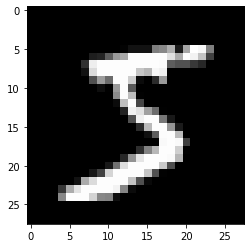In :
``````image, label = dataset
print('image.shape:', image.shape)
plt.imshow(image.permute(1, 2, 0), cmap='gray')
print('Label:', label)``````
```image.shape: torch.Size([1, 28, 28]) Label: 5 ```Next, let's use the `random_split` helper function to set aside 10000 images for our validation set.

In :
``````val_size = 10000
train_size = len(dataset) - val_size

train_ds, val_ds = random_split(dataset, [train_size, val_size])
len(train_ds), len(val_ds)``````
Out:
``(50000, 10000)``

We can now create PyTorch data loaders for training and validation.

In :
``batch_size=128``
In :
``````train_loader = DataLoader(train_ds, batch_size, shuffle=True, num_workers=4, pin_memory=True)

Can you figure out the purpose of the arguments `num_workers` and `pin_memory`? Try looking into the documentation: https://pytorch.org/docs/stable/data.html .

Let's visualize a batch of data in a grid using the `make_grid` function from `torchvision`. We'll also use the `.permute` method on the tensor to move the channels to the last dimension, as expected by `matplotlib`.

In :
``````for images, _ in train_loader:
print('images.shape:', images.shape)
plt.figure(figsize=(16,8))
plt.axis('off')
plt.imshow(make_grid(images, nrow=16).permute((1, 2, 0)))
break``````
```images.shape: torch.Size([128, 1, 28, 28]) ```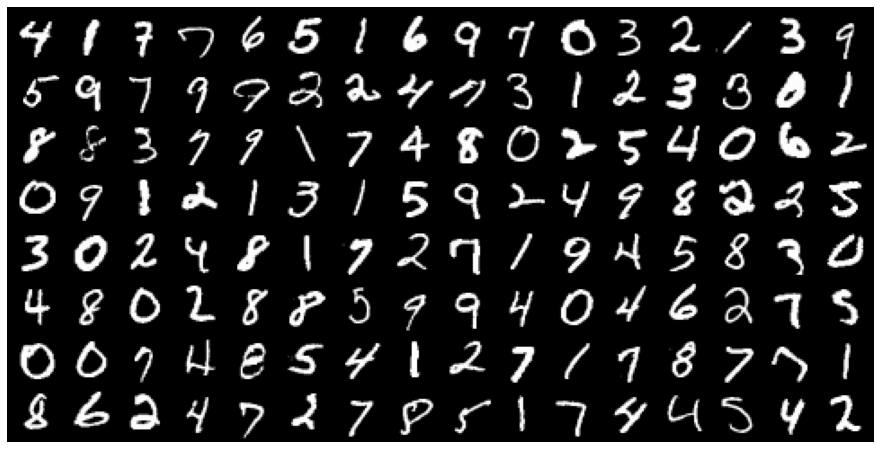### Hidden Layers, Activation Functions and Non-Linearity

We'll create a neural network with two layers: a hidden layer and an output layer. Additionally, we'll use an activation function between the two layers. Let's look at a step-by-step example to learn how hidden layers and activation functions can help capture non-linear relationships between inputs and outputs.

First, let's create a batch of inputs tensors. We'll flatten the `1x28x28` images into vectors of size `784`, so they can be passed into an `nn.Linear` object.

In :
``````for images, labels in train_loader:
print('images.shape:', images.shape)
inputs = images.reshape(-1, 784)
print('inputs.shape:', inputs.shape)
break``````
```images.shape: torch.Size([128, 1, 28, 28]) inputs.shape: torch.Size([128, 784]) ```

Next, let's create a `nn.Linear` object, which will serve as our hidden layer. We'll set the size of the output from the hidden layer to 32. This number can be increased or decreased to change the learning capacity of the model.

In :
``````input_size = inputs.shape[-1]
hidden_size = 32``````
In :
``layer1 = nn.Linear(input_size, hidden_size)``

We can now compute intermediate outputs for the batch of images by passing `inputs` through `layer1`.

In :
``inputs.shape``
Out:
``torch.Size([128, 784])``
In :
``````layer1_outputs = layer1(inputs)
print('layer1_outputs.shape:', layer1_outputs.shape)``````
```layer1_outputs.shape: torch.Size([128, 32]) ```

The image vectors of size `784` are transformed into intermediate output vectors of length `32` by performing a matrix multiplication of `inputs` matrix with the transposed weights matrix of `layer1` and adding the bias. We can verify this using `torch.allclose`. For a more detailed explanation, review the tutorial on linear regression.

In :
``````layer1_outputs_direct = inputs @ layer1.weight.t() + layer1.bias
layer1_outputs_direct.shape
``````
Out:
``torch.Size([128, 32])``
In :
``torch.allclose(layer1_outputs, layer1_outputs_direct, 1e-3)``
Out:
``True``

Thus, `layer1_outputs` and `inputs` have a linear relationship, i.e., each element of `layer_outputs` is a weighted sum of elements from `inputs`. Thus, even as we train the model and modify the weights, `layer1` can only capture linear relationships between `inputs` and `outputs`.Next, we'll use the Rectified Linear Unit (ReLU) function as the activation function for the outputs. It has the formula `relu(x) = max(0,x)` i.e. it simply replaces negative values in a given tensor with the value 0. ReLU is a non-linear function, as seen here visually:We can use the `F.relu` method to apply ReLU to the elements of a tensor.

In :
``````F.relu(torch.tensor([[1, -1, 0],
[-0.1, .2, 3]]))``````
Out:
``````tensor([[1.0000, 0.0000, 0.0000],
[0.0000, 0.2000, 3.0000]])``````

Let's apply the activation function to `layer1_outputs` and verify that negative values were replaced with 0.

In :
``````relu_outputs = F.relu(layer1_outputs)
print('min(layer1_outputs):', torch.min(layer1_outputs).item())
print('min(relu_outputs):', torch.min(relu_outputs).item())``````
```min(layer1_outputs): -0.637366771697998 min(relu_outputs): 0.0 ```

Now that we've applied a non-linear activation function, `relu_outputs` and `inputs` do not have a linear relationship. We refer to `ReLU` as the activation function, because for each input certain outputs are activated (those with non-zero values) while others turned off (those with zero values)

Next, let's create an output layer to convert vectors of length `hidden_size` in `relu_outputs` into vectors of length 10, which is the desired output of our model (since there are 10 target labels).

In :
``````output_size = 10
layer2 = nn.Linear(hidden_size, output_size)
``````
In :
``````layer2_outputs = layer2(relu_outputs)
print(layer2_outputs.shape)
``````
```torch.Size([128, 10]) ```
In :
``inputs.shape``
Out:
``torch.Size([128, 784])``

As expected, `layer2_outputs` contains a batch of vectors of size 10. We can now use this output to compute the loss using `F.cross_entropy` and adjust the weights of `layer1` and `layer2` using gradient descent.

In :
``F.cross_entropy(layer2_outputs, labels)``
Out:
``tensor(2.3167, grad_fn=<NllLossBackward>)``

Thus, our model transforms `inputs` into `layer2_outputs` by applying a linear transformation (using `layer1`), followed by a non-linear activation (using `F.relu`), followed by another linear transformation (using `layer2`). Let's verify this by re-computing the output using basic matrix operations.

In :
``````# Expanded version of layer2(F.relu(layer1(inputs)))
outputs = (F.relu(inputs @ layer1.weight.t() + layer1.bias)) @ layer2.weight.t() + layer2.bias
``````
In :
``torch.allclose(outputs, layer2_outputs, 1e-3)``
Out:
``True``

Note that `outputs` and `inputs` do not have a linear relationship due to the non-linear activation function `F.relu`. As we train the model and adjust the weights of `layer1` and `layer2`, we can now capture non-linear relationships between the images and their labels. In other words, introducing non-linearity makes the model more powerful and versatile. Also, since `hidden_size` does not depend on the dimensions of the inputs or outputs, we vary it to increase the number of parameters within the model. We can also introduce new hidden layers and apply the same non-linear activation after each hidden layer.

The model we just created is called a neural network. A deep neural network is simply a neural network with one or more hidden layers. In fact, the Universal Approximation Theorem states that a sufficiently large & deep neural network can compute any arbitrary function i.e. it can learn rich and complex non-linear relationships between inputs and targets. Here are some examples:

• Identifying if an image contains a cat or a dog (or something else)
• Identifying the genre of a song using a 10-second sample
• Classifying movie reviews as positive or negative based on their content
• Navigating self-driving cars using a video feed of the road
• Translating sentences from English to French (and hundreds of other languages)
• Converting a speech recording to text and vice versa
• And many more...

It's hard to imagine how the simple process of multiplying inputs with randomly initialized matrices, applying non-linear activations, and adjusting weights repeatedly using gradient descent can yield such astounding results. Deep learning models often contain millions of parameters, which can together capture far more complex relationships than the human brain can comprehend.

If we hadn't included a non-linear activation between the two linear layers, the final relationship between inputs and outputs would still be linear. A simple refactoring of the computations illustrates this.

In :
``````# Same as layer2(layer1(inputs))
outputs2 = (inputs @ layer1.weight.t() + layer1.bias) @ layer2.weight.t() + layer2.bias
``````
In :
``````# Create a single layer to replace the two linear layers
combined_layer = nn.Linear(input_size, output_size)

combined_layer.weight.data = layer2.weight @ layer1.weight
combined_layer.bias.data = layer1.bias @ layer2.weight.t() + layer2.bias
``````
In :
``````# Same as combined_layer(inputs)
outputs3 = inputs @ combined_layer.weight.t() + combined_layer.bias
``````
In :
``torch.allclose(outputs2, outputs3, 1e-3)``
Out:
``True``

Whether you're running this Jupyter notebook online or on your computer, it's essential to save your work from time to time. You can continue working on a saved notebook later or share it with friends and colleagues to let them execute your code. Jovian offers an easy way of saving and sharing your Jupyter notebooks online.

In :
``````# Install the library
``````
In :
``import jovian``
In :
``jovian.commit(project='04-feedforward-nn')``
```[jovian] Attempting to save notebook.. [jovian] Updating notebook "aakashns/04-feedforward-nn" on https://jovian.ai/ [jovian] Uploading notebook.. [jovian] Capturing environment.. [jovian] Committed successfully! https://jovian.ai/aakashns/04-feedforward-nn ```
Out:
``'https://jovian.ai/aakashns/04-feedforward-nn'``

`jovian.commit` uploads the notebook to your Jovian account, captures the Python environment, and creates a shareable link for your notebook, as shown above. You can use this link to share your work and let anyone (including you) run your notebooks and reproduce your work.

### Model

We are now ready to define our model. As discussed above, we'll create a neural network with one hidden layer. Here's what that means:

• Instead of using a single `nn.Linear` object to transform a batch of inputs (pixel intensities) into outputs (class probabilities), we'll use two `nn.Linear` objects. Each of these is called a layer in the network.

• The first layer (also known as the hidden layer) will transform the input matrix of shape `batch_size x 784` into an intermediate output matrix of shape `batch_size x hidden_size`. The parameter `hidden_size` can be configured manually (e.g., 32 or 64).

• We'll then apply a non-linear activation function to the intermediate outputs. The activation function transforms individual elements of the matrix.

• The result of the activation function, which is also of size `batch_size x hidden_size`, is passed into the second layer (also known as the output layer). The second layer transforms it into a matrix of size `batch_size x 10`. We can use this output to compute the loss and adjust weights using gradient descent.

As discussed above, our model will contain one hidden layer. Here's what it looks like visually:Let's define the model by extending the `nn.Module` class from PyTorch.

In :
``````class MnistModel(nn.Module):
"""Feedfoward neural network with 1 hidden layer"""
def __init__(self, in_size, hidden_size, out_size):
super().__init__()
# hidden layer
self.linear1 = nn.Linear(in_size, hidden_size)
# output layer
self.linear2 = nn.Linear(hidden_size, out_size)

def forward(self, xb):
# Flatten the image tensors
xb = xb.view(xb.size(0), -1)
# Get intermediate outputs using hidden layer
out = self.linear1(xb)
# Apply activation function
out = F.relu(out)
# Get predictions using output layer
out = self.linear2(out)
return out

def training_step(self, batch):
images, labels = batch
out = self(images)                  # Generate predictions
loss = F.cross_entropy(out, labels) # Calculate loss
return loss

def validation_step(self, batch):
images, labels = batch
out = self(images)                    # Generate predictions
loss = F.cross_entropy(out, labels)   # Calculate loss
acc = accuracy(out, labels)           # Calculate accuracy
return {'val_loss': loss, 'val_acc': acc}

def validation_epoch_end(self, outputs):
batch_losses = [x['val_loss'] for x in outputs]
epoch_loss = torch.stack(batch_losses).mean()   # Combine losses
batch_accs = [x['val_acc'] for x in outputs]
epoch_acc = torch.stack(batch_accs).mean()      # Combine accuracies
return {'val_loss': epoch_loss.item(), 'val_acc': epoch_acc.item()}

def epoch_end(self, epoch, result):
print("Epoch [{}], val_loss: {:.4f}, val_acc: {:.4f}".format(epoch, result['val_loss'], result['val_acc']))``````

We also need to define an `accuracy` function which calculates the accuracy of the model's prediction on an batch of inputs. It's used in `validation_step` above.

In :
``````def accuracy(outputs, labels):
_, preds = torch.max(outputs, dim=1)

We'll create a model that contains a hidden layer with 32 activations.

In :
``````input_size = 784
hidden_size = 32 # you can change this
num_classes = 10``````
In :
``model = MnistModel(input_size, hidden_size=32, out_size=num_classes)``

Let's take a look at the model's parameters. We expect to see one weight and bias matrix for each of the layers.

In :
``````for t in model.parameters():
print(t.shape)
``````
```torch.Size([32, 784]) torch.Size() torch.Size([10, 32]) torch.Size() ```

Let's try and generate some outputs using our model. We'll take the first batch of 128 images from our dataset and pass them into our model.

In :
``````for images, labels in train_loader:
outputs = model(images)
loss = F.cross_entropy(outputs, labels)
print('Loss:', loss.item())
break

print('outputs.shape : ', outputs.shape)
print('Sample outputs :\n', outputs[:2].data)``````
```Loss: 2.342543363571167 outputs.shape : torch.Size([128, 10]) Sample outputs : tensor([[ 0.0861, -0.1378, -0.2982, 0.2218, -0.1095, 0.0333, -0.2329, 0.1517, -0.2202, 0.0442], [ 0.1395, -0.0609, -0.2785, 0.2629, -0.1903, 0.0455, -0.2295, 0.1441, -0.2312, -0.0005]]) ```

### Using a GPU

As the sizes of our models and datasets increase, we need to use GPUs to train our models within a reasonable amount of time. GPUs contain hundreds of cores optimized for performing expensive matrix operations on floating-point numbers quickly, making them ideal for training deep neural networks. You can use GPUs for free on Google Colab and Kaggle or rent GPU-powered machines on services like Google Cloud Platform, Amazon Web Services, and Paperspace.

We can check if a GPU is available and the required NVIDIA CUDA drivers are installed using `torch.cuda.is_available`.

In :
``torch.cuda.is_available()``
Out:
``False``

Let's define a helper function to ensure that our code uses the GPU if available and defaults to using the CPU if it isn't.

In :
``````def get_default_device():
"""Pick GPU if available, else CPU"""
if torch.cuda.is_available():
else:
In :
``````device = get_default_device()
device
``````
Out:
``device(type='cpu')``

Next, let's define a function that can move data and model to a chosen device.

In :
``````def to_device(data, device):
"""Move tensor(s) to chosen device"""
if isinstance(data, (list,tuple)):
return [to_device(x, device) for x in data]
return data.to(device, non_blocking=True)``````
In :
``````for images, labels in train_loader:
print(images.shape)
images = to_device(images, device)
print(images.device)
break``````
```torch.Size([128, 1, 28, 28]) cpu ```

Finally, we define a `DeviceDataLoader` class to wrap our existing data loaders and move batches of data to the selected device. Interestingly, we don't need to extend an existing class to create a PyTorch datal oader. All we need is an `__iter__` method to retrieve batches of data and an `__len__` method to get the number of batches.

In :
``````class DeviceDataLoader():
"""Wrap a dataloader to move data to a device"""
def __init__(self, dl, device):
self.dl = dl
self.device = device

def __iter__(self):
"""Yield a batch of data after moving it to device"""
for b in self.dl:
yield to_device(b, self.device)

def __len__(self):
"""Number of batches"""
return len(self.dl)``````

The `yield` keyword in Python is used to create a generator function that can be used within a `for` loop, as illustrated below.

In :
``````def some_numbers():
yield 10
yield 20
yield 30

for value in some_numbers():
print(value)
``````
```10 20 30 ```

We can now wrap our data loaders using `DeviceDataLoader`.

In :
``````train_loader = DeviceDataLoader(train_loader, device)
``````

Tensors moved to the GPU have a `device` property which includes that word `cuda`. Let's verify this by looking at a batch of data from `valid_dl`.

In :
``````for xb, yb in val_loader:
print('xb.device:', xb.device)
print('yb:', yb)
break``````
```xb.device: cpu yb: tensor([6, 6, 4, 3, 4, 4, 7, 0, 6, 9, 2, 9, 7, 1, 3, 2, 5, 8, 7, 0, 5, 4, 4, 1, 9, 8, 3, 6, 9, 5, 0, 6, 7, 0, 6, 2, 2, 1, 9, 9, 8, 9, 0, 8, 5, 4, 1, 8, 1, 1, 3, 4, 6, 2, 1, 8, 1, 0, 7, 4, 6, 2, 3, 3, 7, 3, 6, 0, 8, 3, 0, 9, 2, 4, 6, 8, 9, 4, 8, 6, 2, 5, 7, 8, 1, 5, 2, 5, 3, 0, 5, 9, 1, 7, 4, 6, 0, 5, 9, 4, 7, 5, 0, 4, 0, 9, 5, 1, 9, 2, 3, 9, 3, 5, 7, 4, 6, 9, 3, 9, 8, 9, 3, 2, 1, 7, 0, 5, 1, 8, 9, 9, 2, 4, 3, 3, 5, 1, 4, 5, 7, 8, 5, 9, 4, 7, 5, 7, 4, 1, 1, 4, 1, 2, 7, 2, 4, 0, 0, 9, 7, 4, 9, 8, 4, 9, 4, 2, 7, 9, 6, 7, 1, 7, 3, 3, 5, 1, 5, 3, 4, 6, 2, 1, 6, 9, 2, 0, 1, 4, 2, 5, 0, 4, 0, 7, 9, 7, 7, 0, 9, 1, 7, 8, 8, 6, 2, 4, 5, 8, 4, 6, 6, 1, 5, 5, 0, 9, 3, 9, 0, 5, 0, 4, 1, 7, 9, 6, 0, 3, 2, 6, 8, 8, 0, 5, 3, 2, 3, 6, 5, 4, 1, 1, 5, 8, 1, 0, 3, 3, 5, 1, 4, 4, 0, 8]) ```

### Training the Model

We'll define two functions: `fit` and `evaluate` to train the model using gradient descent and evaluate its performance on the validation set. For a detailed walkthrough of these functions, check out the previous tutorial.

In :
``````def evaluate(model, val_loader):
"""Evaluate the model's performance on the validation set"""
outputs = [model.validation_step(batch) for batch in val_loader]
return model.validation_epoch_end(outputs)

"""Train the model using gradient descent"""
history = []
optimizer = opt_func(model.parameters(), lr)
for epoch in range(epochs):
# Training Phase
loss = model.training_step(batch)
loss.backward()
optimizer.step()
# Validation phase
model.epoch_end(epoch, result)
history.append(result)
return history``````

Before we train the model, we need to ensure that the data and the model's parameters (weights and biases) are on the same device (CPU or GPU). We can reuse the `to_device` function to move the model's parameters to the right device.

In :
``````# Model (on GPU)
model = MnistModel(input_size, hidden_size=hidden_size, out_size=num_classes)
to_device(model, device)
``````
Out:
``````MnistModel(
(linear1): Linear(in_features=784, out_features=32, bias=True)
(linear2): Linear(in_features=32, out_features=10, bias=True)
)``````

Let's see how the model performs on the validation set with the initial set of weights and biases.

In :
``````history = [evaluate(model, val_loader)]
history
``````
Out:
``[{'val_loss': 2.3129286766052246, 'val_acc': 0.12646484375}]``

The initial accuracy is around 10%, as one might expect from a randomly initialized model (since it has a 1 in 10 chance of getting a label right by guessing randomly).

Let's train the model for five epochs and look at the results. We can use a relatively high learning rate of 0.5.

In :
``history += fit(5, 0.5, model, train_loader, val_loader)``
```Epoch , val_loss: 0.2544, val_acc: 0.9197 Epoch , val_loss: 0.1827, val_acc: 0.9480 Epoch , val_loss: 0.2222, val_acc: 0.9311 Epoch , val_loss: 0.1479, val_acc: 0.9551 Epoch , val_loss: 0.1317, val_acc: 0.9602 ```

96% is pretty good! Let's train the model for five more epochs at a lower learning rate of 0.1 to improve the accuracy further.

In :
``history += fit(5, 0.1, model, train_loader, val_loader)``
```Epoch , val_loss: 0.1093, val_acc: 0.9674 Epoch , val_loss: 0.1083, val_acc: 0.9670 Epoch , val_loss: 0.1088, val_acc: 0.9660 Epoch , val_loss: 0.1069, val_acc: 0.9687 Epoch , val_loss: 0.1075, val_acc: 0.9684 ```

We can now plot the losses & accuracies to study how the model improves over time.

In :
``````losses = [x['val_loss'] for x in history]
plt.plot(losses, '-x')
plt.xlabel('epoch')
plt.ylabel('loss')
plt.title('Loss vs. No. of epochs');``````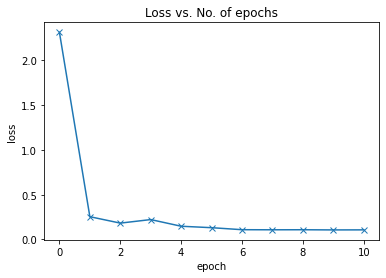In :
``````accuracies = [x['val_acc'] for x in history]
plt.plot(accuracies, '-x')
plt.xlabel('epoch')
plt.ylabel('accuracy')
plt.title('Accuracy vs. No. of epochs');``````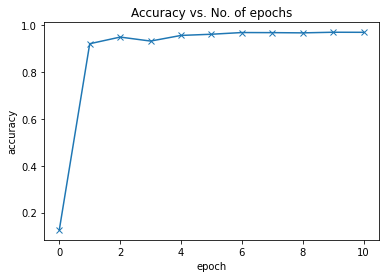Our current model outperforms the logistic regression model (which could only achieve around 86% accuracy) by a considerable margin! It quickly reaches an accuracy of 97% but doesn't improve much beyond this. To improve accuracy further, we need to make the model more powerful by increasing the hidden layer's size or adding more hidden layers with activations. I encourage you to try out both these approaches and see which one works better.

As a final step, we can save and commit our work using the `jovian` library.

In :
``!pip install jovian --upgrade -q``
In :
``import jovian``
In :
``jovian.commit(project='04-feedforward-nn', environment=None)``
```[jovian] Attempting to save notebook.. [jovian] Updating notebook "aakashns/04-feedforward-nn" on https://jovian.ai/ [jovian] Uploading notebook.. [jovian] Committed successfully! https://jovian.ai/aakashns/04-feedforward-nn ```
Out:
``'https://jovian.ai/aakashns/04-feedforward-nn'``

### Testing with individual images

While we have been tracking the overall accuracy of a model so far, it's also a good idea to look at model's results on some sample images. Let's test out our model with some images from the predefined test dataset of 10000 images. We begin by recreating the test dataset with the `ToTensor` transform.

In :
``````# Define test dataset
test_dataset = MNIST(root='data/',
train=False,
transform=ToTensor())
``````

Let's define a helper function `predict_image`, which returns the predicted label for a single image tensor.

In :
``````def predict_image(img, model):
xb = to_device(img.unsqueeze(0), device)
yb = model(xb)
_, preds  = torch.max(yb, dim=1)
return preds.item()``````

Let's try it out with a few images.

In :
``````img, label = test_dataset
plt.imshow(img, cmap='gray')
print('Label:', label, ', Predicted:', predict_image(img, model))``````
```Label: 7 , Predicted: 7 ```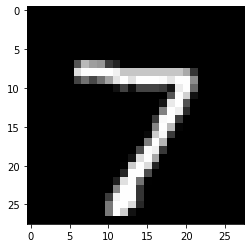In :
``````img, label = test_dataset
plt.imshow(img, cmap='gray')
print('Label:', label, ', Predicted:', predict_image(img, model))``````
```Label: 2 , Predicted: 2 ```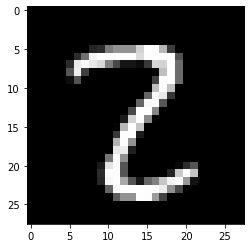In :
``````img, label = test_dataset
plt.imshow(img, cmap='gray')
print('Label:', label, ', Predicted:', predict_image(img, model))``````
```Label: 9 , Predicted: 9 ```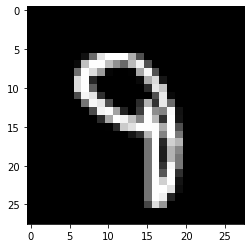Identifying where our model performs poorly can help us improve the model, by collecting more training data, increasing/decreasing the complexity of the model, and changing the hypeparameters.

As a final step, let's also look at the overall loss and accuracy of the model on the test set.

In :
``````test_loader = DeviceDataLoader(DataLoader(test_dataset, batch_size=256), device)
result
``````
Out:
``{'val_loss': 0.09472835808992386, 'val_acc': 0.971484363079071}``

We expect this to be similar to the accuracy/loss on the validation set. If not, we might need a better validation set that has similar data and distribution as the test set (which often comes from real world data).

Let's save the model's weights and attach it to the notebook using `jovian.commit`. We will also record the model's performance on the test dataset using `jovian.log_metrics`.

In :
``jovian.log_metrics(test_loss=result['val_loss'], test_acc=result['val_loss'])``
```[jovian] Metrics logged. ```
In :
``torch.save(model.state_dict(), 'mnist-feedforward.pth')``
In [ ]:
``````jovian.commit(project='04-feedforward-nn',
environment=None,
outputs=['mnist-feedforward.pth'])``````
```[jovian] Attempting to save notebook.. ```

### Exercises

Try out the following exercises to apply the concepts and techniques you have learned so far:

Training great machine learning models reliably takes practice and experience. Try experimenting with different datasets, models and hyperparameters, it's the best way to acquire this skill.

Here is a summary of the topics covered in this tutorial:

• We created a neural network with one hidden layer to improve upon the logistic regression model from the previous tutorial. We also used the ReLU activation function to introduce non-linearity into the model, allowing it to learn more complex relationships between the inputs (pixel densities) and outputs (class probabilities).

• We defined some utilities like `get_default_device`, `to_device` and `DeviceDataLoader` to leverage a GPU if available, by moving the input data and model parameters to the appropriate device.

• We were able to use the exact same training loop: the `fit` function we had define earlier to train out model and evaluate it using the validation dataset.

There's a lot of scope to experiment here, and I encourage you to use the interactive nature of Jupyter to play around with the various parameters. Here are a few ideas:

• Try changing the size of the hidden layer, or add more hidden layers and see if you can achieve a higher accuracy.

• Try changing the batch size and learning rate to see if you can achieve the same accuracy in fewer epochs.

• Compare the training times on a CPU vs. GPU. Do you see a significant difference. How does it vary with the size of the dataset and the size of the model (no. of weights and parameters)?

• Try building a model for a different dataset, such as the CIFAR10 or CIFAR100 datasets.

Here are some references for further reading:

You are now ready to move on to the next tutorial: Image Classification using Convolutional Neural Networks.